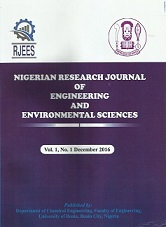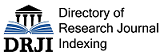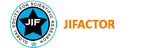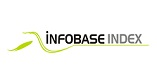### Bending Analysis of a Three-Dimensional Isotropic Thick Plate using Energy Method with Trigonometric Displacement Functions

Authors: Onyeka FC, Nwa-David CD, Sule J

DOI Info: http://doi.org/10.5281/zenodo.6724573

#### ABSTRACT

This paper applied a trigonometric function to the study of exact bending analysis of a three-dimensional isotropic rectangular thick plate that is clamped at the first-three edges and the other edge simply supported (CCCS). This function was developed by making use of the three-dimensional (3-D) static theory of elasticity which consist of entire stress components used in the total potential energy equation formulation. The compatibility equation which was built from the energy equation transformation which was used to get the slope and deflection relationship. The solution of compatibility equation gave rise to the exact trigonometric deflection function while the coefficient of deflection of the plate was obtained from the governing equation through direct variation method. These solutions were applied in the analysis of the bending problem of the CCCS rectangular plate by establishing the formula for calculating the displacement and stresses of the plate. The result of this study showed that the value of the deflection and stresses decrease as the span-thickness ratio increases. The comparative study shows that the present study differs with classical plate theory (CPT) and refined plate theory (RPT) of assumed deflection by 5.6% and 4.9% respectively, whereas it differs from the exact 2-D RPT by 3.7%. This showed that the 3-D plate theory is a sufficient model for the plate analysis, which produced an exact solution to the bending problem of any category of rectangular plate under CCCS boundary condition.

Affiliations: Department of Civil Engineering, Edo State University, Uzairue, Edo State, Nigeria.
Keywords: Exact Bending Analysis, 3-D Plate Theory, Isotropic Thick Plate, Energy Method, Trigonometric Displacement Function
Published date: 2022/06/30##### SUBMIT A MANUSCRIPTISSN: 2635-3342 (Print)

ISSN: 2635-3350 (Online)

DOI: In progress

ISI Impact Factor: In progress

##### Indexing & Abstracting(+234) 806 927 5563

Chemical Engineering Department, Faculty of Engineering, University of Benin, PMB 1154, Ugbowo, Benin City, Edo State, Nigeria.